# Dense rational distance sets in the plane

 Importance: High ✭✭✭
 Author(s): Ulam, Stanislaw M.
 Subject: Geometry
 Keywords: integral distance rational distance
 Posted by: mdevos on: July 4th, 2008
Problem   Does there exist a dense set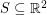so that all pairwise distances between points inare rational?

This famous problem was asked by Ulam, who guessed the answer would be negative.

A cute theorem of Erdos shows that ifis non-collinear and all pairwise distances between points inare integral, thenis finite. For the proof, first note that if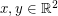have distance, then every point which has integer distance to bothand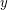must lie on one of thehyperbolas consisting of those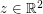with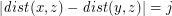for some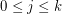. So, if all pairwise distances between points inare integral, andare non-collinear, then every other point inmust lie on an intersection between one of finitely many hyperbola with foci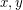and one of finitely many with foci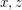. This set is necessarily finite, thus completing the proof.

Of course, the above argument gives no upper bound on the size of a non-collinear set of points inwith pairwise integral distances. Indeed, if Ulam's conjecture is true, then there exist such sets of arbitrary size. Surprisingly, it is very difficult to construct such setsof even rather small size. Recently Kreisel and Kurz [KK] found such a set of size 7, but it is unknown if there exists one of size 8.

It is trivial to find infinitely many points on a line with all pairwise distances rational. Less trivially, there exist infinite subsets of a circle with all pairwise distances rational. Very recently, Solymosi and De Zeeuw [SZ] proved that these are the only two irreducible algebraic curves with this property. This suggests that, if the answer to Ulam's problem is affirmative, such a setmust be extremely special.

## Bibliography

[KK] T. Kreisel and S. Kurz, There are integral heptagons, no three points on a line, no four on a circle, Discrete & Computational Geometry, Online first: DOI 10.1007/s00454-007-9038-6

[SZ] J. Solymosi and F. de Zeeuw, On a question of Erdos and Ulam.

* indicates original appearance(s) of problem.